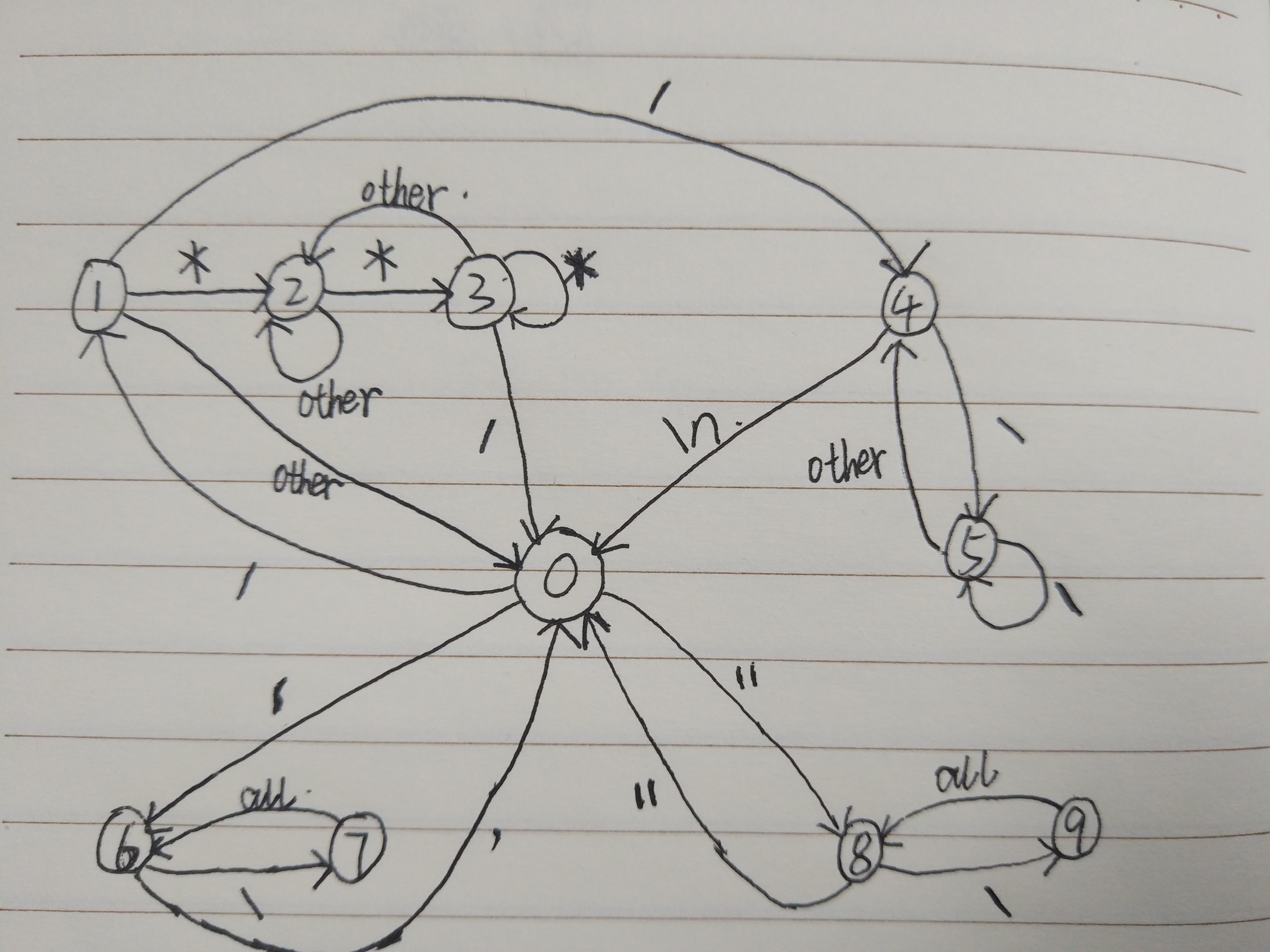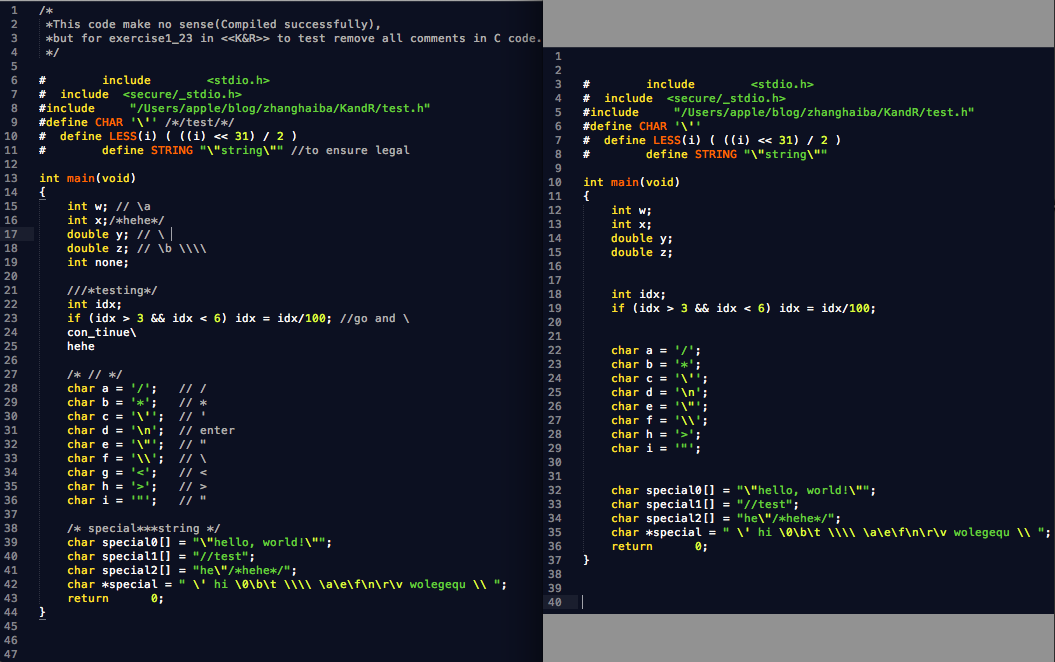# 怎样删除C/C++代码中的所有注释？浅谈状态机的编程思想

K&R习题1-23中，要求“编写一个程序，删除C语言程序中所有的注释语句。要正确处理带引号的字符串与字符常量。在C语言中，注释不允许嵌套”

【一个简单的状态机】

（1）当前状态为OUT：若当前字符是空白字符(空格、制表符、换行符），则维护当前状态仍为OUT；否则改变状态为IN。

（2）当前状态为IN：若遇到的当前字符是非空白字符，则维护当前状态为IN；否则改变状态为OUT。

#include <stdio.h>
#define OUT 0 /* outside a word */
#define IN 1  /* inside a word  */
int main(void)
{
int c, state;
state = OUT;
while ( ( c = getchar() ) != EOF ) {
if (state == OUT) {
if (c == ' ' || c == '\t' || c == '\n')
state = OUT;
else {
state = IN;
putchar(c); //action
}
} else {
if (c != ' ' && c != '\t' && c != '\n') {
state = IN;
putchar(c); //action
} else {
putchar('\n');//action
state = OUT;
}
}
}
return 0;
}

【“编写一个程序，删除C语言程序中所有的注释语句。要正确处理带引号的字符串与字符常量。在C语言中，注释不允许嵌套”】

00）设正常状态为0，并初始为正常状态

01）状态0中遇到/，说明可能会遇到注释，则进入状态1　　　　　　　　　　ex. int a = b; /

02）状态1中遇到*，说明进入多行注释部分，则进入状态2　　　　　　　　　ex. int a= b; /*

03）状态1中遇到/，说明进入单行注释部分，则进入状态4　　　　　　　　　ex. int a = b; //

04）状态1中没有遇到*或/，说明/是路径符号或除号，则恢复状态0　　　　  ex. <secure/_stdio.h> or 5/3

05）状态2中遇到*，说明多行注释可能要结束，则进入状态3　　　　　　　　ex. int a = b; /*heh*

06）状态2中不是遇到*，说明多行注释还在继续，则维持状态2　　　　　　　ex. int a = b; /*hehe

07）状态3中遇到/，说明多行注释要结束，则恢复状态0　　　　　　　　　　ex. int a = b; /*hehe*/

08）状态3中遇到*，则恢复状态3　　　　　　　　　　                           ex. int a = b; /***

09）状态3中不是遇到/或*，说明多行注释只是遇到*，恢复状态2 　            ex. int a = b; /*hehe*h

10）状态4中遇到\，说明可能进入折行注释部分，则进入状态5　　　　　　　ex. int a = b; //hehe\

11）状态5中遇到\，说明可能进入折行注释部分，则维护状态5　　　　　　　ex. int a = b; //hehe\\\

12）状态5中遇到其它字符，则说明进入了折行注释部分，则恢复状态4　　　 ex. int a = b; // hehe\a or hehe\<enter>

13）状态4中遇到回车符\n，说明单行注释结束，则恢复状态0 　　　　　　　ex. int a = b; //hehe<enter>

14）状态0中遇到'，说明进入字符常量中，则进入状态6 　　　　　　　　　　ex. char a = '

15）状态6中遇到\，说明遇到转义字符，则进入状态7　　　　　　　　　　　ex. char a = '\

16）状态7中遇到任何字符，都恢复状态6 　　　　　　　　　　　　　　　　ex. char a = '\n 还有如'\t', '\'', '\\' 等 主要是防止'\''，误以为结束

17）状态6中遇到'，说明字符常量结束，则进入状态0　　　　 　　　　　　　ex. char a = '\n'

18）状态0中遇到"，说明进入字符串常量中，则进入状态8　　　　　　　　　ex. char s[] = "

19）状态8中遇到\，说明遇到转义字符，则进入状态9　　　　　　　　　　　ex. char s[] = "\

20）状态9中遇到任何字符，都恢复状态8　　　　　　　　　　　　　　　 　ex. char s[] = "\n 主要是防止"\"，误以为结束

21）状态8中遇到"字符，说明字符串常量结束，则恢复状态0　　　　　　　　ex. char s[] = "\"hehe"#include<stdio.h>
int main()
{
char c;
int state=0;
freopen("1.in","r",stdin);
freopen("1.out","w",stdout);
while((c=getchar())!=EOF)
{
switch(state)
{
case 0:
if(c=='/')// ex. [/]
state=1;
else if(c=='\'')// ex. [']
state=6;
else if(c=='\"')// ex. ["]
state=8;
else
putchar(c);
break;
case 1:
if(c=='*')// ex. [/*]
state=2;
else if(c=='/')// ex. [//]
state=4;
else
{ // ex. [<secure/_stdio.h> or 5/3]
putchar('/');
putchar(c);
state=0;
}
break;
case 2:
if(c=='*') // ex. [/*he*]
state=3;
else // ex. [/*heh]
state=2;
break;
case 3:
if(c=='/')// ex. [/*heh*/]
state=0;
else if(c=='*')
state=3;//ex. [/***]注意这里，不加这个条件，*的个数是奇数的时候出错
else// ex. [/*heh*e]
state=2;
break;
case 4:
if(c=='\\')// ex. [//hehe\]
state=5;
else if(c=='\n')// ex. [//hehe<enter>]
{
state=0;
putchar(c);
}
break;
case 5:
if(c=='\\')// ex. [//hehe\\\\\]
state=5;
else// ex. [//hehe\<enter> or //hehe\a]
state=4;
break;
case 6:
if(c=='\\')// ex. ['\]
state=7;
else if(c=='\'')// ex. ['\n' or '\'' or '\t' ect.]
{
state=0;
putchar(c);
}
break;
case 7:// ex. ['\n or '\' or '\t etc.]
state=6;
break;
case 8:
if(c=='\\')// ex. ["\]
state=9;
else if(c=='\"')// ex. ["\n" or "\"" or "\t" ect.]
{
state=0;
putchar(c);
}
break;
case 9:// ex. ["\n or "\" or "\t ect.]
state=8;
break;
}
if(state==6||state==7||state==8||state==9)
putchar(c);
}
return 0;
}


【测试用例（1）a.out < test.c > test2.c】
test.c如下：

/*
*This code make no sense(Compiled successfully),
*but for exercise1_23 in <<K&R>> to test remove all comments in C code.
*/

#          include         <stdio.h>
#  include  <secure/_stdio.h>
#include      "/Users/apple/blog/zhanghaiba/KandR/test.h"
#define CHAR '\'' /*/test/*/
#  define LESS(i) ( ((i) << 31) / 2 )
#        define STRING "\"string\"" //to ensure legal

int main(void)
{
int w; // \a
int x;/*hehe*/
double y; // \
double z; // \b \\\\
int none;

///*testing*/
int idx;
if (idx > 3 && idx < 6) idx = idx/100; //go and \
con_tinue\
hehe

/* // */
char a = '/';    // /
char b = '*';    // *
char c = '\'';    // '
char d = '\n';    // enter
char e = '\"';    // "
char f = '\\';    // \
char g = '<';    // <
char h = '>';    // >
char i = '"';    // "

/* special***string */
char special0[] = "\"hello, world!\"";
char special1[] = "//test";
char special2[] = "he\"/*hehe*/";
char *special = " \' hi \0\b\t \\\\ \a\e\f\n\r\v wolegequ \\ ";
return        0;
}

（说明：由于编辑器高亮的原因，注意//后面加字符\的折行注释部分颜色其实不对的，另外17行\后面是有一个空格的）【测试用例（2）./a.out < ~/open_src_code/glibc-2.17/malloc/malloc.c > test2.c】

glibc-2.17源码中的的malloc.c包括空行和注释有5163行，经过上面去注释后代码变成3625行。

06-0807-30
06-15
03-20
08-18248
10-303147
11-181万+
02-176172
01-149515
08-263930
02-26487
06-24535
09-272518
07-293731
11-074
05-091761
05-2340
07-151611
12-0135
©️2020 CSDN 皮肤主题: 大白 设计师:CSDN官方博客